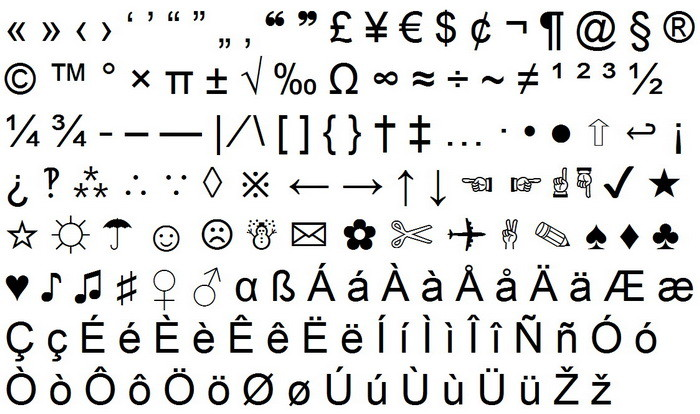# List Of Keyboard Shortcuts To Add Fancy Symbol TitlesBelow you will find a list of the ALT keyboard shortcuts to create symbols. If you are using a Microsoft Windows PC computer, you can also use the following to make it even simpler.

Go To: Computer > All programs > Accessories > System Tools > Character Map.

At the top of that window scroll down to “areal Unicode MS”.

On an empty place on that window right click and click on “pin to start menu”.

Alt + 0153 = ™ trademark symbol

Alt + 0174 = ® registered trademark symbol

Alt + 0176 = ° degree symbol

Alt + 0177 = ± plus or minus sign

Alt + 0182 = ¶ paragraph mark

Alt + 0190 = ¾ fraction three fourths

Alt + 0215 = × multiplication sign

Alt + 0162 = ¢ the cent sign

Alt + 0161 = ¡ upside down exclamation point

Alt + 0191 = ¿ upside down question mark

Alt + 1 = ☺ smiley face

Alt + 2 = ☻ black smiley face

Alt + 15 = ☼ sun

Alt + 12 = ♀ female sign

Alt + 11 = ♂ male sign

Alt + 6 = ♠ spade

Alt + 5 = ♣ club

Alt + 3 = ♥ heart

Alt + 4 = ♦ diamond

Alt + 13 = ♪ eighth note

Alt + 14 = ♫ beamed eighth note

Alt + 8721 = ∑ nary summation (auto sum)

Alt + 251 = √ square root check mark

Alt + 8236 = ∞ infinity

Alt + 24 = ↑ up arrow

Alt + 25 = ↓ down arrow

Alt + 26 = → right arrow

Alt + 27 = ← left arrow

Alt + 18 = ↕ up/down arrow

Alt + 29 = ↔ left right arrow